# Class 9 RD Sharma Solutions – Chapter 13 Linear Equation in Two Variable – Exercise 13.3 | Set 2

### Question 11: Draw the graph of the equation 2x + 3y = 12. From the graph, find the coordinates of the point:

(i) whose y-coordinates is 3.

(ii) whose x-coordinate is −3.

Solution:

Given:

2x + 3y = 12

We get,Substituting x = 0 in y =we get,y = 4

Substituting x = 6 in, we gety = 4

Hence, we have the following table exhibiting the abscissa and ordinates of points on the line represented by the given equation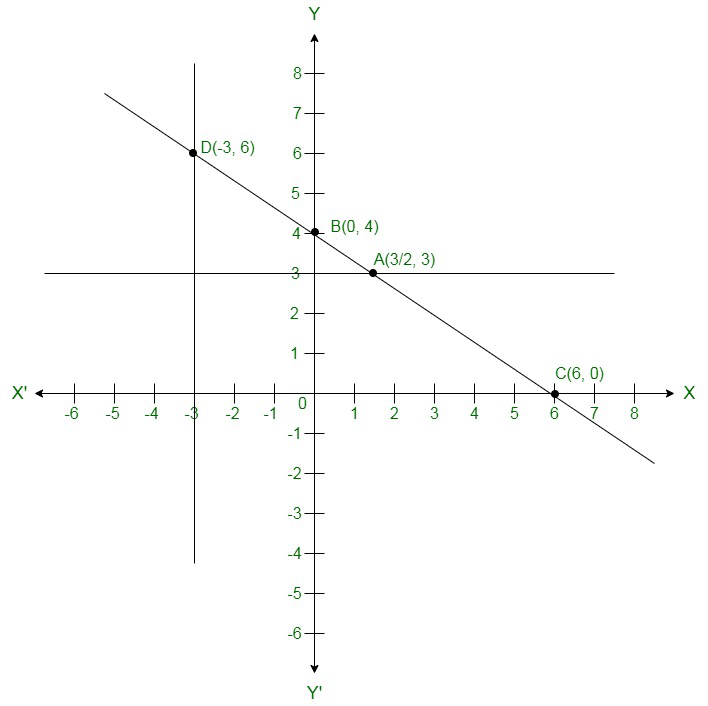By plotting the given equation on the graph, we get the point B (0, 4) and C (6,0).

(i) Co-ordinates of the point whose y axis is 3 are A(ii) Co-ordinates of the point whose x -coordinate is –3 are D (-3, 6)

### Question 12: Draw the graph of each of the equations given below. Also, find the coordinates of the points where the graph cuts the coordinate axes:

(i) 6x − 3y = 12

(ii) −x + 4y = 8

(iii) 2x + y = 6

(iv) 3x + 2y + 6 = 0

Solution:

(i) Given:

6x – 3y = 12

We get,Now, substituting x = 0 in, we get

y = -4

Substituting x = 2 in, we get

y = 0

Hence, we have the following table exhibiting the abscissa and ordinates of points on the line represented by the given equation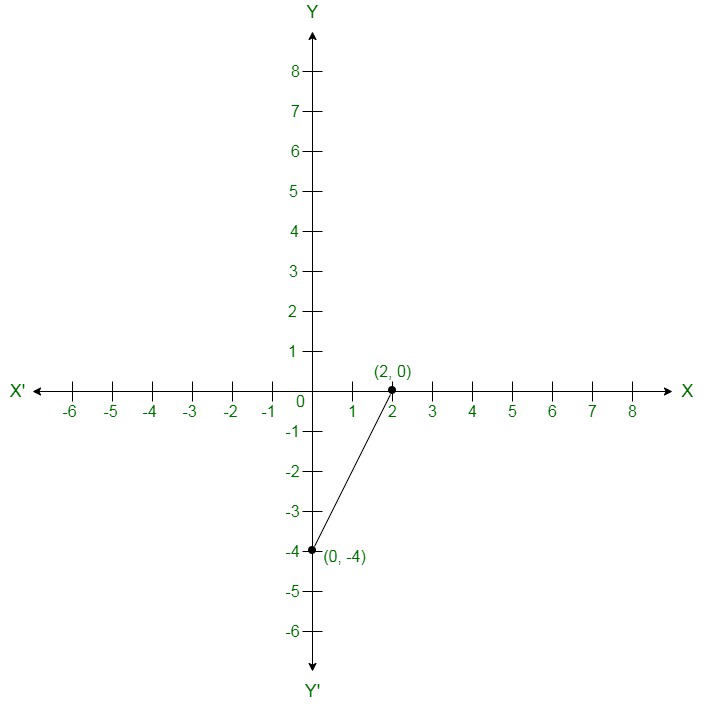Co-ordinates of the points where graph cuts the co-ordinate axes are at y = -4 axis and x = 2

at x axis.

(ii) Given:

-x + 4y = 8

We get,Now, substituting x = 0 in, we get

y = 2

Substituting x = -8 in, we get

y = 0

Hence, we have the following table exhibiting the abscissa and ordinates of points on the line represented by the given equation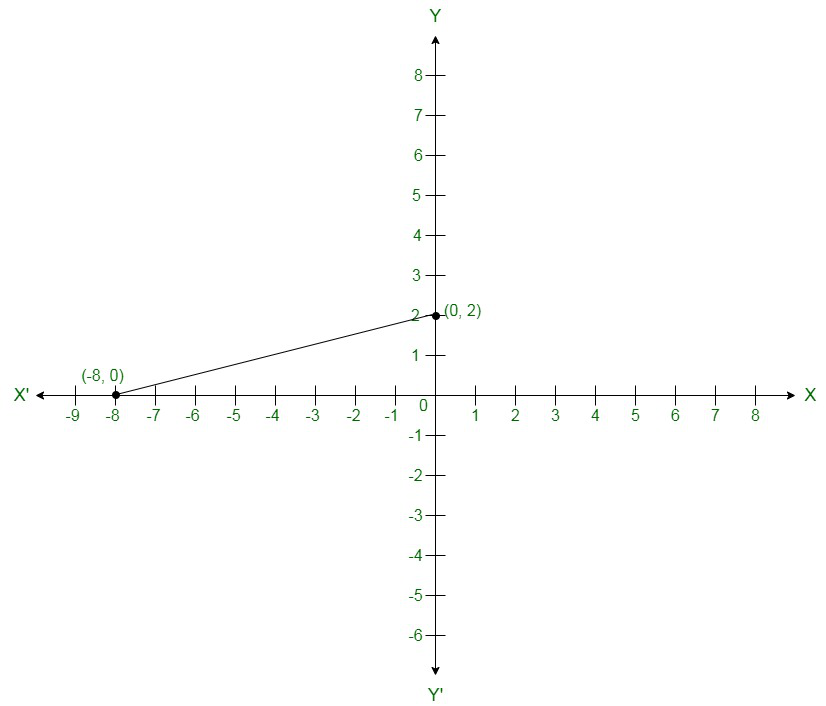Co-ordinates of the points where graph cuts the co-ordinate axes are at y = 2 axis and x = -8

at x axis.

(iii) Given:

2x + y = 6

We get,

y = 6 – 2x

Now, substituting x= 0 in y = 6 – 2x w e get

y = 6

Substituting x = 3 in y = 6 – 2x, we get

y = 0

Hence, we have the following table exhibiting the abscissa and ordinates of points on the line represented by the given equation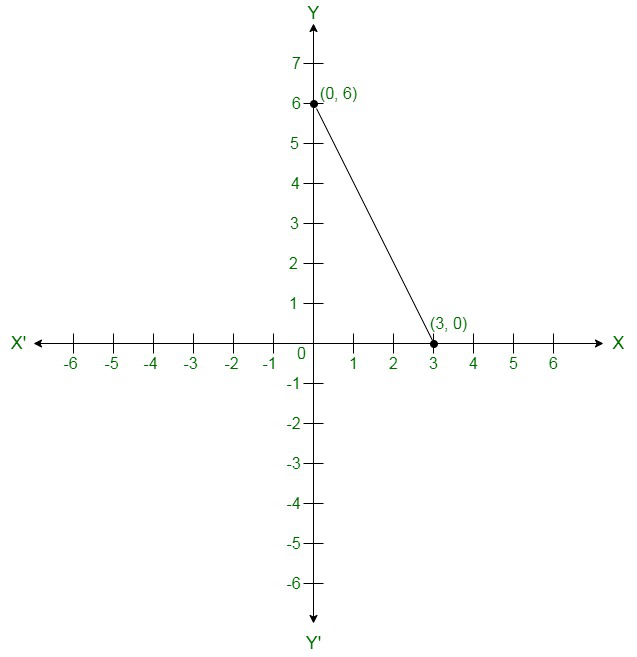Co-ordinates of the points where graph cuts the co-ordinate axes are y = 6 at y axis and x = 3

at x axis.

(iv) Given:

3x + 2y + 6 = 0

We get,Now, substituting x = -2 in, we get

y = -3

Substituting x = -2 in, we get

y = 0

Hence, we have the following table exhibiting the abscissa and ordinates of points on the line represented by the given equation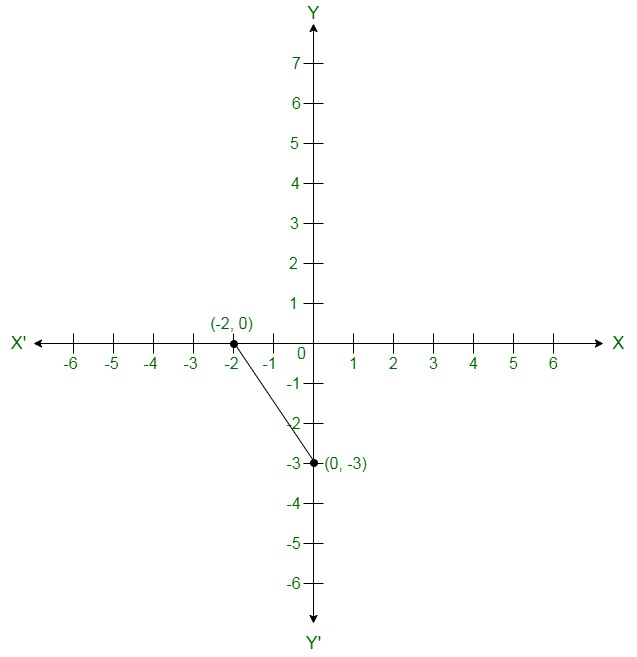Co-ordinates of the points where graph cuts the co-ordinate axes are y = -3 at y axis and x = -2

at x axis.

### Question 13: Draw the graph of the equation 2x + y = 6. Shade the region bounded by the graph and the coordinate axes. Also, find the area of the shaded region.

Solution:

Given:

2x + y = 6

We get,

y = 6 – 2 x

Now, substituting x = 0 in y = 6 – 2x ,we get

y = 6

Substituting x = 3 in y = 6 – 2x ,we get

Hence, we have the following table exhibiting the abscissa and ordinates of points on the line represented by the given equation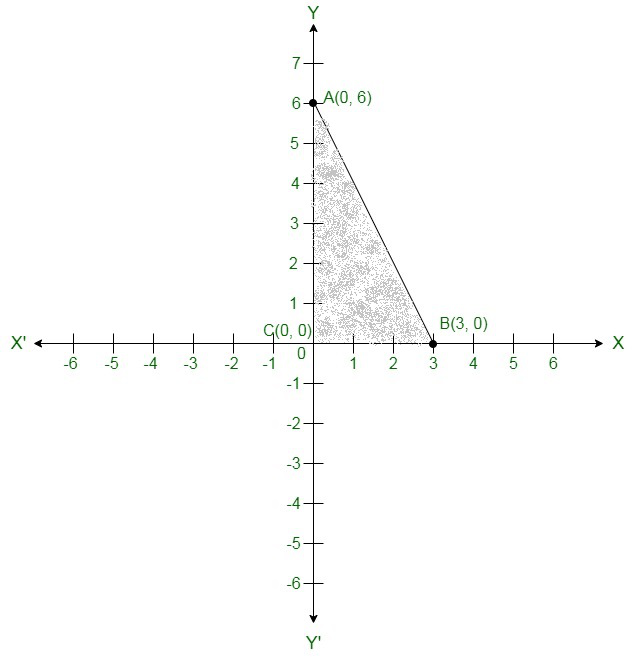The region bounded by the graph is ABC which forms a triangle.

AC at y axis is the base of triangle having AC = 6 units on y axis.

BC at x axis is the height of triangle having BC = 3 units on x axis.

Therefore,

Area of triangle ABC, say A is given byA = 9 sq. units

### Question 14: Draw the graph of the equation. Also, find the area of the triangle formed by the line and the coordinates axes.

Solution:

Given:4x + 3y = 12

We get,Now, substituting x = 0 in, we get

y = 4

Substituting x = 3 in, we get

y = 0

Hence, we have the following table exhibiting the abscissa and ordinates of points on the line represented by the given equation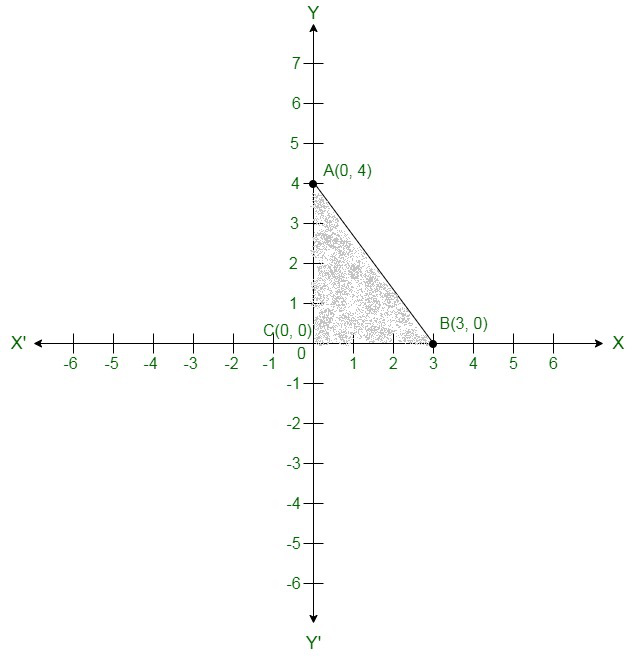The region bounded by the graph is ABC which form a triangle.

AC at y axis is the base of triangle having AC = 4 units on y axis.

BC at x axis is the height of triangle having BC = 3 units on x axis.

Therefore,

Area of triangle ABC, say A is given byA = 6 sq. units

### Question 15: Draw the graph of y = | x |.

Solution:

We are given,

y = |x|

Substituting, x = 1 we get

y = 1

Substituting, x = -1 we get

y = 1

Substituting,x = 2 we get

y = 2

Substituting, x = -2 we get

y = 2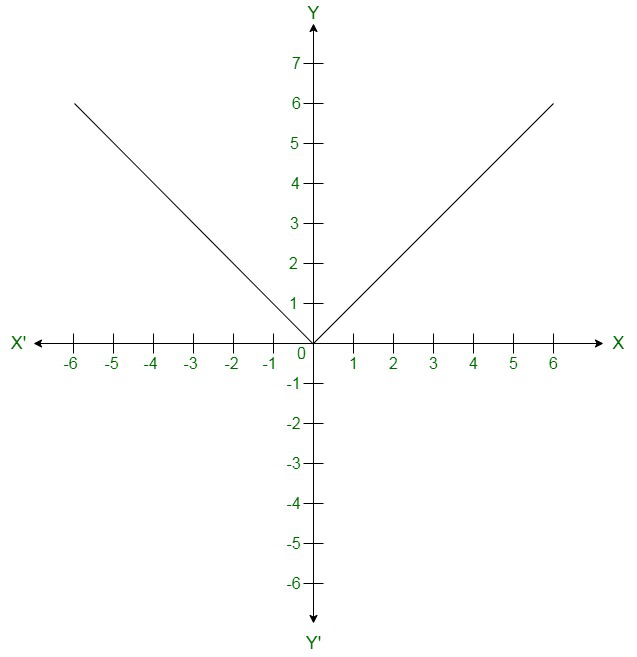For every value of x, whether positive or negative, we get y as a positive number.

### Question 16: Draw the graph of y = | x | + 2.

Solution:

Given:

y = |x| + 2

Substituting, x = 0 we get

y = 2

Substituting, x = 1 we get

y = 3

Substituting, x = -1 we get

y = 3

Substituting, x = 2 we get

y = 4

Substituting, x = -2 we get

y = 4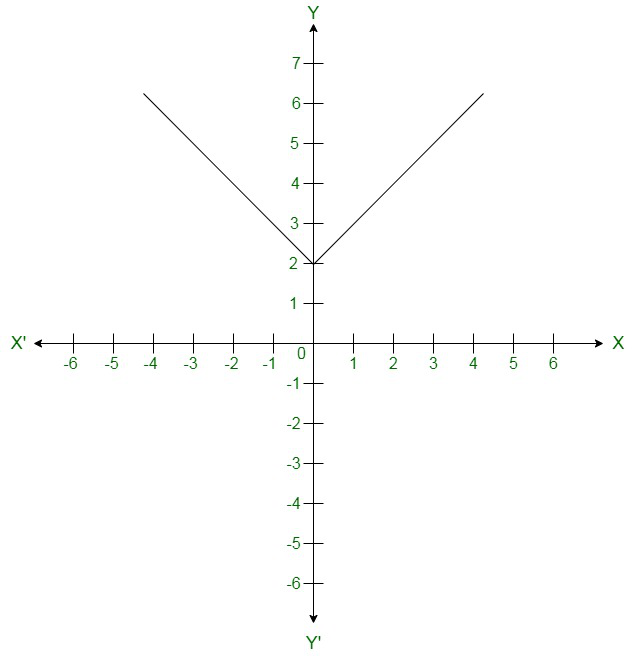For every value of x, whether positive or negative, we get y as a positive number and the minimum value of y is equal to 2 units.

### Question 17: Draw the graphs of the following linear equations on the same graph paper:

2x + 3y = 12, x − y = 1

### Find the coordinates of the vertices of the triangle formed by the two straight lines and the area bounded by these lines and x-axis.

Solution:

Given:

2x + 3y = 12

We get,Now, substituting x = 0  in, we get

y = 4

Substituting x = 6 in, we get

y = 0

Therefore, we have the following table exhibiting the abscissa and ordinates of points on the line represented by the given equation

Plotting A(0,4) and E(6,0) on the graph and by joining the points , we obtain the graph of equation .

Given:

x – y = 1

We get,

y = x − 1

Now, substituting x = 0 in y = x − 1,we get

y = −1

Substituting x = -1  in y = x − 1,we get

y = −2

Therefore, we have the following table exhibiting the abscissa and ordinates of points on the line represented by the given equation

Plotting D(0,1) and E(-1,0) on the graph and by joining the points , we obtain the graph of equation .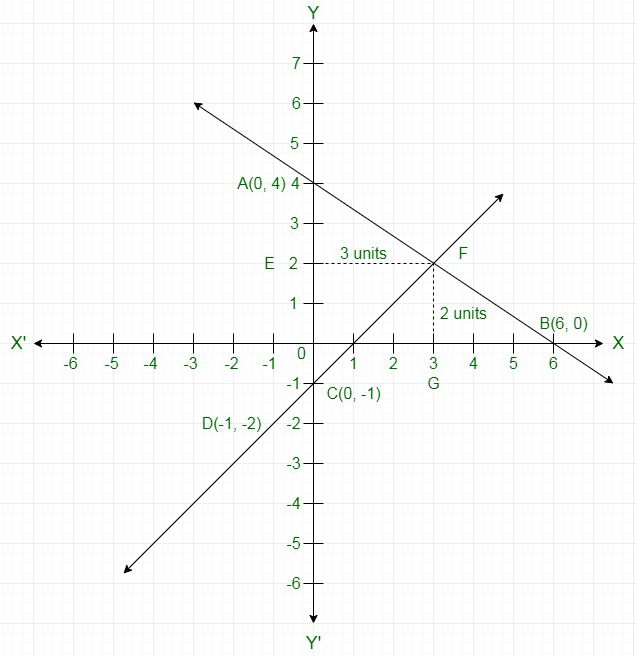By the intersection of lines formed by 2x + 3y = 12 and x – y = 1 on the graph, triangle ABC is formed on y axis.

Therefore,

AC at y axis is the base of triangle ABC having AC = 5 units on y axis.

Draw FE perpendicular from F on y axis.

FE parallel to x axis is the height of triangle ABC having FE = 3 units on x axis.

Therefore,

Area of triangle ABC, say A is given by### Question 18: Draw the graphs of the linear equations 4x − 3y + 4 = 0 and 4x + 3y −20 = 0. Find the area bounded by these lines and x-axis.

Solution:

Given:

4x – 3y + 4 = 0

We get,Now, substituting x = 0 in, we getSubstituting x = -1 in, we get

y = 0

Hence, we have the following table exhibiting the abscissa and ordinates of points on the line represented by the given equation

Plotting E(0,) and A(-1,0) on the graph and by joining the points, we obtain the graph of equation.

We are given,

4x + 3y – 20 = 0

We get,Now, substituting x = 0 in, we getSubstituting x = 5 in, we get

y = 0

Therefore, we have the following table exhibiting the abscissa and ordinates of points on the line represented by the given equation

Plotting D(0,) and B(5,0) on the graph and by joining the points, we obtain the graph of equation.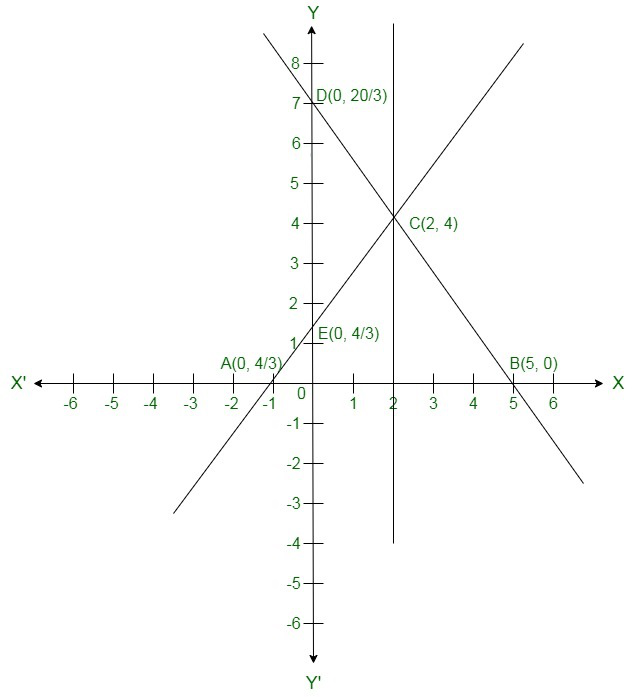By the intersection of lines formed by 4x – 3y + 4 = 0 and 4x + 3y – 20 = 0 on the graph, triangle ABC is formed on x axis.

Therefore,

AB at x axis is the base of triangle ABC having AB = 6 units on x axis.

Draw CF perpendicular from C on x axis.

CF parallel to y axis is the height of triangle ABC having CF = 4 units on y axis.

Therefore,

Area of triangle ABC, say A is given by### Question 19: The path of a train A is given by the equation 3x + 4y − 12 = 0 and the path of another train B is given by the equation 6x + 8y − 48 = 0. Represent this situation graphically.

Solution:

Given: Path of train A,

3x + 4y – 12 = 0

We get,Now, substituting x = 0 in,we get

y = 3

Substituting x = 4 in,we get

y = 0

Therefore, we have the following table exhibiting the abscissa and ordinates of points on the line represented by the given equation

Plotting A(4,0) and E(0,3) on the graph and by joining the points , we obtain the graph of equation .

We are given the path of train B,

6x + 8y – 48 = 0

We get,Now, substituting x = 0 in,we get

y = 6

Substituting x = 8 in,we get

y = 0

Therefore, we have the following table exhibiting the abscissa and ordinates of points on the line represented by the given equation

Plotting C(0,6) and D(8,0) on the graph and by joining the points , we obtain the graph of equation.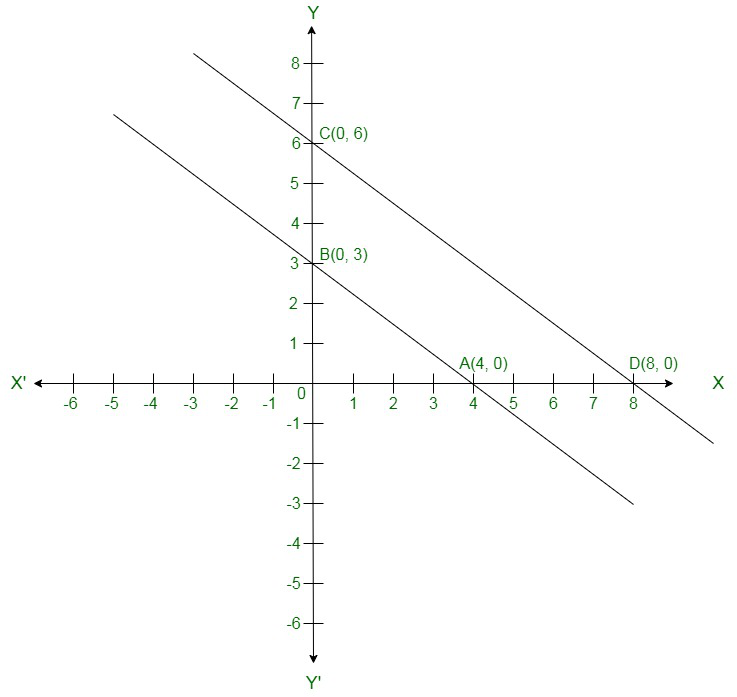### Question 20: Ravish tells his daughter Aarushi, ”Seven years ago, I was seven times as old as you were then. Also, three years from now, I shall be three times as old as you will be”. If present ages of Aarushi and Ravish are x and y years respectively, represent this situation algebraically as well as graphically.

Solution:

We are given the present age of Ravish as y years and Aarushi as x years.

Age of Ravish seven years ago = y – 7

Age of Aarushi seven years ago = x – 7

It has already been said by Ravish that seven years ago he was seven times old then Aarushi was then

So,

y – 7 = 7(x – 7)

y – 7 = 7x – 49

7x – y = -7 + 49

7x – y – 42 = 0

Age of Ravish three years from now = y + 3

Age of Aarushi three years from now = x + 3

It has already been said by Ravish that three years from now he will be three times old then Aarushi will be then

So,

y + 3 = 3(x + 3)

y + 3 = 3x + 9

3x + 9 – y – 3 = 0

3x – y + 6 = 0

(1) and (2) are the algebraic representation of the given statement.

Given:

7x – y – 42 = 0

We get,

y = 7x – 42

Now, substituting x = 0 in y = 7x – 42 ,we get

y = -42

Substituting x = 6 in y = 7x – 42, we get

y = 0

Therefore, we have the following table exhibiting the abscissa and ordinates of points on the line represented by the given equation

Given:

3x – y + 6 = 0

We get,

y = 3x + 6

Now, substituting x = 0 in y = 3x + 6 ,we get

y = 6

Substituting x = -2 in y = 3x + 6 ,we get

y = 0

Therefore, we have the following table exhibiting the abscissa and ordinates of points on the line represented by the given equation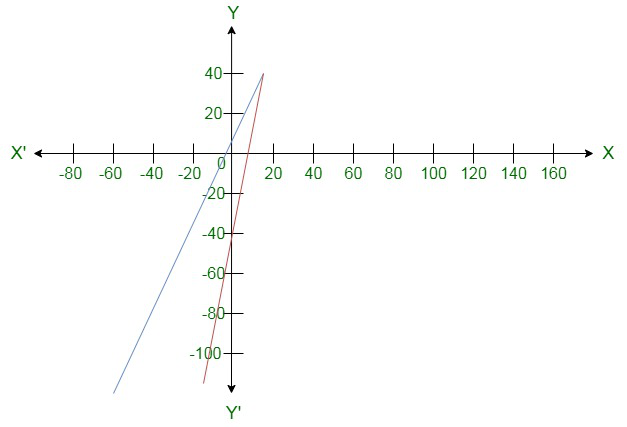The red -line represents the equation. 7x – y – 42 = 0

The blue-line represents the equation. 3x – y + 6 = 0

### Question 21: Aarushi was driving a car with uniform speed of 60km/h. Draw distance-time graph. From the graph, find the distance traveled by Aarushi in

(i)Hours

(ii)Hour

Solution:

Aarushi is driving the car with the uniform speed of 60 km/h.

We represent time on X-axis and distance on Y-axis

Now, graphically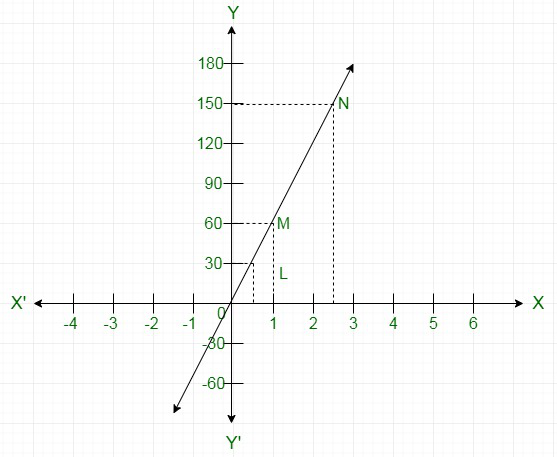We are given that the car is travelling with a uniform speed 60 km/hr. This means car travels 60 km distance each hour. Thus the graph we get is of a straight line.

Also, we know when the car is at rest, the distance traveled is 0 km, speed is 0 km/hr and the time is also 0 hr.

Therefore, the given straight line will pass through O(0,0) and M(1,60).

Join the points O and M and extend the line in both directions.

Now, we draw a dotted line parallel to y-axis from x =that meets the straight line graph at L from which we draw a line parallel to x-axis that crosses y-axis at 30. Thus, inhr, distance traveled by the car is 30 km.

Now, we draw a dotted line parallel to y-axis from x =that meets the straight line graph at N from which we draw a line parallel to x-axis that crosses y-axis at 150. Thus, inhr, distance traveled by the car is 150 km.

(i) Distance = Speed × Time

Distance traveled inhours is given by

Distance =Distance =Distance = 150 km

(ii) Distance = Speed × Time

Distance traveled inhours is given by

Distance =Distance = 30 km

Whether you're preparing for your first job interview or aiming to upskill in this ever-evolving tech landscape, GeeksforGeeks Courses are your key to success. We provide top-quality content at affordable prices, all geared towards accelerating your growth in a time-bound manner. Join the millions we've already empowered, and we're here to do the same for you. Don't miss out - check it out now!

Previous
Next